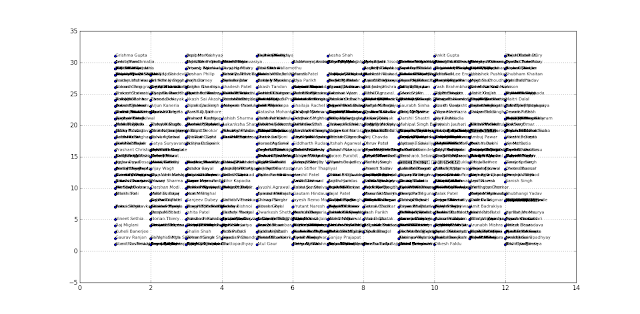So today I exported the Facebook Calendar Data for birthdays from my account. To acquire this information, users can go to this  and click on Birthdays. To download the file, just change the url protocol from webcal to http.linkOnce we have the ics file with us exported from Facebook, I used python to parse the content. Installed the following libraries using pip: iCalendar, Matplotlib and networkx ( didn't implement but experiementing ). Here's the python code for you.

```from icalendar import Calendar, Event
from datetime import datetime
info = []
x_list = []
y_list = []
labels = []
dts = []
sorted_info = []
g = open('u100006338374486.ics','rb')
gcal = Calendar.from_ical(g.read())
for component in gcal.walk():

if component.name == "VEVENT":

dt = component.get('DTSTART').dt

dt = dt.replace(year = 1000)

info.append([dt,component.get('SUMMARY').encode('utf8')])

x_list.append(dt.month)

y_list.append(dt.day)

dts.append(dt)

labels.append(component.get('SUMMARY').encode('utf8'))
g.close()
info.sort(key=lambda r: r)
#sorted birthday information
import matplotlib.pyplot as plt
#import networkx as nx
#G=nx.Graph()
fig = plt.figure()
ax = fig.add_subplot(111)
plt.scatter(x_list,y_list)
counter=0
for i,j in zip(x_list,y_list):

ax.annotate(labels[counter].decode('utf8')[0:labels[counter].decode('utf8').find("'")],(i,j),fontsize=8)

counter+=1
plt.grid()
plt.show()
plt.savefig("example.png")```

Executing the script, I got the following output. I am thinking of getting a bigger data set or doing some neural network prediction with this dataset. Too ambitious, perhaps, but we have to start somewhere right? Suggestion  to FBOpenSource Devs, please make an API for generating this data.